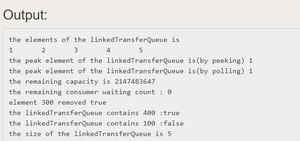# Java Program to Implement LinkedTransferQueue API

• Difficulty Level : Medium
• Last Updated : 27 Apr, 2021

LinkedTransferQueue is a queue that orders elements FIFO (first-in-first-out) with respect to any given producer. The head of the queue is that element that has been on the queue the longest time for some producer. The tail of the queue is that element that has been on the queue the shortest time for some producer.

Constructor Summary

2. LinkedTransferQueue​(Collection<? extends E> c): Creates a LinkedTransferQueue initially containing the elements of the given collection, added in traversal order of the collection’s iterator.

## Java

 `// Java Program to Implement LinkedTransferQueue API` `import` `java.util.Collection;``import` `java.util.Iterator;``import` `java.util.concurrent.LinkedTransferQueue;``import` `java.util.concurrent.TimeUnit;` `public` `class` `LinkedTransferQueueImpl {``    ``private` `LinkedTransferQueue linkedTransferQueue;` `    ``// Create an empty LinkedTransferQueue``    ``public` `LinkedTransferQueueImpl()``    ``{``        ``linkedTransferQueue = ``new` `LinkedTransferQueue();``    ``}` `    ``// Creates a LinkedTransferQueue containing``    ``// the elements of the given collection``    ``public` `LinkedTransferQueueImpl(``        ``Collection c)``    ``{``        ``linkedTransferQueue = ``new` `LinkedTransferQueue(c);``    ``}` `    ``// add the given element at the end or tail``    ``// of queue``    ``public` `boolean` `add(E e)``    ``{``        ``return` `linkedTransferQueue.add(e);``    ``}` `    ``// remove all the elements from queue``    ``public` `void` `clear() { linkedTransferQueue.clear(); }` `    ``// return true if the given object is present``    ``public` `boolean` `contains(Object o)``    ``{``        ``return` `linkedTransferQueue.contains(o);``    ``}` `    ``// Returns an estimate of the number of consumers``    ``// waiting to receive elements via BlockingQueue.take()``    ``// or timed poll.``    ``public` `int` `getWaitingConsumerCount()``    ``{``        ``return` `linkedTransferQueue``            ``.getWaitingConsumerCount();``    ``}` `    ``// Returns true if there is at least one consumer``    ``// waiting to receive an element via``    ``// BlockingQueue.take() or timed poll.``    ``public` `boolean` `hasWaitingConsumer()``    ``{``        ``return` `linkedTransferQueue.hasWaitingConsumer();``    ``}` `    ``// remove all the elements from the queue and add them``    ``// to the provided Collection``    ``public` `int` `drainTo(Collection c)``    ``{``        ``return` `linkedTransferQueue.drainTo(c);``    ``}` `    ``// Removes at most the given number of available``    ``// elements from this queue and adds them to the given``    ``// collection.``    ``public` `int` `drainTo(Collection c,``                       ``int` `maxElements)``    ``{``        ``return` `linkedTransferQueue.drainTo(c, maxElements);``    ``}` `    ``// Returns an iterator over the elements in this queue``    ``// in proper sequence.``    ``public` `Iterator iterator()``    ``{``        ``return` `linkedTransferQueue.iterator();``    ``}` `    ``// Inserts the specified element at the tail of this``    ``// queue if it is possible to do so immediately without``    ``// exceeding the queue's capacity, returning true upon``    ``// success and false if this queue is full.``    ``public` `boolean` `offer(E e)``    ``{``        ``return` `linkedTransferQueue.offer(e);``    ``}` `    ``// Inserts the specified element at the tail of this``    ``// queue, waiting up to the specified wait time for``    ``// space to become available if the queue is full.``    ``public` `boolean` `offer(E e, ``long` `timeout, TimeUnit unit)``        ``throws` `InterruptedException``    ``{``        ``return` `linkedTransferQueue.offer(e, timeout, unit);``    ``}` `    ``// Retrieves, but does not remove, the head of this``    ``// queue, or returns null if this queue is empty.``    ``public` `E peek() { ``return` `linkedTransferQueue.peek(); }` `    ``// Retrieves and removes the head of this queue, or``    ``// returns null if this queue is empty.``    ``public` `E poll() { ``return` `linkedTransferQueue.poll(); }` `    ``// Retrieves and removes the head of this queue, waiting``    ``// up to the specified wait time if necessary for an``    ``// element to become available.``    ``public` `E poll(``long` `timeout, TimeUnit unit)``        ``throws` `InterruptedException``    ``{``        ``return` `linkedTransferQueue.poll(timeout, unit);``    ``}` `    ``// Inserts the specified element at the tail of this``    ``// queue, waiting for space to become available if the``    ``// queue is full.``    ``public` `void` `put(E e) ``throws` `InterruptedException``    ``{``        ``linkedTransferQueue.put(e);``    ``}` `    ``// Returns the number of additional elements that this``    ``// queue can ideally (in the absence of memory or``    ``// resource constraints) accept without blocking.``    ``public` `int` `remainingCapacity()``    ``{``        ``return` `linkedTransferQueue.remainingCapacity();``    ``}` `    ``// Removes a single instance of the specified element``    ``// from this queue, if it is present.``    ``public` `boolean` `remove(Object o)``    ``{``        ``return` `linkedTransferQueue.remove(o);``    ``}` `    ``// Returns the number of elements in this queue. **/``    ``public` `int` `size() { ``return` `linkedTransferQueue.size(); }` `    ``// Retrieves and removes the head of this queue, waiting``    ``// if necessary until an element becomes available``    ``public` `E take() ``throws` `InterruptedException``    ``{``        ``return` `linkedTransferQueue.take();``    ``}` `    ``// Returns an array containing all of the elements in``    ``// this queue, in proper sequence.``    ``public` `Object[] toArray()``    ``{``        ``return` `linkedTransferQueue.toArray();``    ``}` `    ``// Returns an array containing all of the elements in``    ``// this queue, in proper sequence; the runtime type of``    ``//  the returned array is that of the specified array.``    ``public` ` T[] toArray(T[] a)``    ``{``        ``return` `linkedTransferQueue.toArray(a);``    ``}` `    ``// Returns a string representation of this collection.``    ``public` `String toString()``    ``{``        ``return` `linkedTransferQueue.toString();``    ``}` `    ``// Transfers the element to a consumer, waiting if``    ``// necessary to do so.``    ``public` `void` `transfer(E e) ``throws` `InterruptedException``    ``{``        ``linkedTransferQueue.transfer(e);``    ``}` `    ``public` `static` `void` `main(String[] args)``    ``{``        ``// create a linkedtransfer queue``        ``LinkedTransferQueueImpl linkedTransferQueue``            ``= ``new` `LinkedTransferQueueImpl();` `        ``try` `{` `            ``// add elements``            ``linkedTransferQueue.put(``"1"``);``            ``linkedTransferQueue.put(``"2"``);``            ``linkedTransferQueue.put(``"3"``);``        ``}``        ``catch` `(InterruptedException e) {``            ``e.printStackTrace();``        ``}``        ``linkedTransferQueue.add(``"4"``);``        ``linkedTransferQueue.add(``"5"``);``        ``System.out.println(``            ``"the elements of the linkedTransferQueue is "``);` `        ``// iterate and print elements``        ``Iterator itr``            ``= linkedTransferQueue.iterator();``        ``while` `(itr.hasNext()) {``            ``System.out.print(itr.next() + ``"\t"``);``        ``}``        ``System.out.println();``        ``linkedTransferQueue.offer(``"6"``);``        ``linkedTransferQueue.offer(``"7"``);``        ``System.out.println(``            ``"the peak element of the linkedTransferQueue is(by peeking) "``            ``+ linkedTransferQueue.peek());``        ``System.out.println(``            ``"the peak element of the linkedTransferQueue is(by polling) "``            ``+ linkedTransferQueue.poll());``        ``System.out.println(``            ``"the remaining capacity is "``            ``+ linkedTransferQueue.remainingCapacity());``        ``System.out.println(``            ``"the remaining consumer waiting count : "``            ``+ linkedTransferQueue``                  ``.getWaitingConsumerCount());``        ``System.out.println(``            ``"element 300 removed "``            ``+ linkedTransferQueue.remove(``"3"``));``        ``System.out.println(``            ``"the linkedTransferQueue contains 400 :"``            ``+ linkedTransferQueue.contains(``"4"``));``        ``System.out.println(``            ``"the linkedTransferQueue contains 100 :"``            ``+ linkedTransferQueue.contains(``"1"``));``        ``System.out.println(``            ``"the size of the linkedTransferQueue is "``            ``+ linkedTransferQueue.size());``        ``System.out.println(linkedTransferQueue);``    ``}``}`My Personal Notes arrow_drop_up